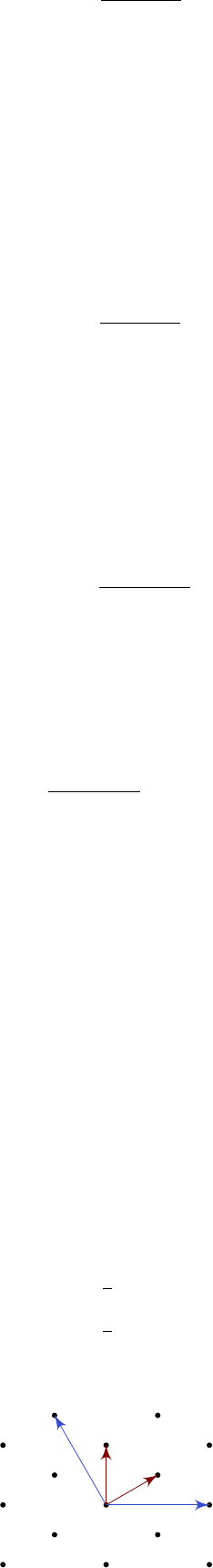6Representation of Lie algebras

III Symmetries, Fields and Particles6.2 Root and weight lattices
Recall that the simple roots are a basis for the whole root space. In particular,
any positive root can be written as a positive sum of simple roots. So for any
β Φ, we can write
β =
r
X
i=1
β
i
α
(i)
,
where β
i
Z for i = 1, ··· , r. Hence all roots lie in a root lattice:
Definition
(Root lattice)
.
Let
g
be a Lie algebra with simple roots
α
(i)
. Then
the root lattice is defined as
L[g] = span
Z
{α
(i)
: i = 1, ··· , r}.
Note that here we are taking the integer span, not the real span.
What we want to do is to define an analogous weight lattice, where all possible
weights lie.
We start by defining some funny normalization of the roots:
Definition (Simple co-root). The simple co-roots are
ˇα
(i)
=
2α
(i)
(α
(i)
, α
(i)
)
.
Similarly, we can define the co-root lattice:
Definition
(Co-root lattice)
.
Let
g
be a Lie algebra with simple roots
α
(i)
.
Then the co-root lattice is defined as
ˇ
L[g] = span
Z
{ˇα
(i)
: i = 1, ··· , r}.
Definition
(Weight lattice)
.
The weight lattice
L
W
[
g
] is the dual to the co-root
lattice:
L
W
[g] =
ˇ
L
[g] = {λ h
R
: (λ, µ) Z for all µ
ˇ
L[g]}.
So we know λ L
W
[g] iff for all α
(i)
, we have
(λ, ˇα
(i)
) =
2(α
(i)
, λ)
(α
(i)
, α
(i)
)
Z.
So by what we have previously computed, we know that all weights
λ S
ρ
are
in L
W
[g].
Given the basis
B = {ˇα
(i)
: i = 1, ··· , r}
for
ˇ
L[g], we define the dual basis
B
= {ω
(i)
: i = 1, ··· , r}
by demanding
(ˇα
(i)
, ω
(j)
) =
2(α
(i)
, ω
(j)
)
(α
(i)
, α
(i)
)
= δ
ij
.
These
ω
(i)
are known as the fundamental weights. As the simple roots span
h
R
,
we can write
ω
(i)
=
r
X
j=1
B
ij
α
(j)
for some B
ij
R. Plugging this into the definition, we find
r
X
k=1
2(α
(i)
, α
(k)
)
(α
(i)
, α
(i)
)
B
jk
= δ
i
j
.
So we know
r
X
k=1
B
jk
A
ki
= δ
i
j
,
i.e. B is the inverse of the Cartan matrix A. Thus we can write
α
(i)
=
r
X
j=1
A
ij
ω
(j)
.
Example. Consider g = A
2
. Then we have
A =
2 1
1 2
.
Then we have
α = α
(1)
= 2ω
(1)
ω
(2)
β = α
(2)
= ω
(1)
+ 2ω
(2)
.
So we have
ω
(1)
=
1
3
(2α + β)
ω
(2)
=
1
3
(α + 2β).
We can then draw the weight lattice:
ω
(2)
ω
(1)
α
β
Note that the roots are also in the weight lattice, because they are weights of
Given any weight λ S
ρ
L
W
[g], we can write
λ =
r
X
i=1
λ
i
ω
(i)
for some λ
i
Z. These {λ
i
} are called Dynkin labels of the weight λ.